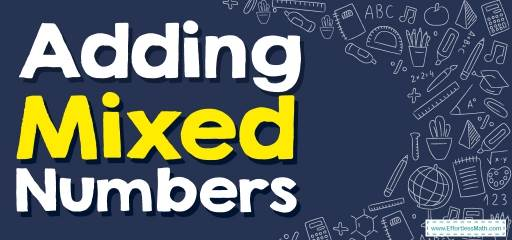# How to Add Mixed Numbers? (+FREE Worksheet!)

Fractions greater than $$1$$ are usually represented as mixed numbers. Join us in this post to learn more about mixed numbers and how to add them.Fractions greater than $$1$$ are usually represented as mixed numbers. In this case, the mixed number consists of an integer part and a standard fraction less than $$1$$. The integer part is the same as the quotient part and the numerator of the fraction is the remainder of the division and the denominator of the fraction will also be the divisor.

## Step by step guide to Adding Mixed Numbers

The addition of mixed numbers is very similar to the addition of integers and has two forms:

1- Adding mixed numbers when their denominators are the same:

Adding mixed numbers when the denominators of fractions are the same is always simple.

• Step 1: Add the integers together.
• Step 2: Add the numerator of the fractions together.
• Step 3: If the sum of fractions becomes an improper fraction, convert it to a mixed number and write the answer.

2- Adding mixed numbers when their denominators are different:

The most difficult type of addition of mixed numbers is when the denominators of the fractions are different.

• Step 1: Add the integers together.
• Step 2: Add the fractions together: In this section, you must first find the Least Common Denominator (LCD), and then you can add the numerator of the fractions together.
• Step 3: If the sum of fractions becomes an improper fraction, convert it to a mixed number and write the answer.

The Absolute Best Book for 5th Grade Students

### Adding Mixed Numbers – Example 1:

Add mixed numbers. $$1 \ \frac{1}{2} \ + \ 2 \ \frac{2}{3}=$$

Solution:

Rewriting our equation with parts separated, $$1+\frac{1}{2}+2+\frac{2}{3}$$,

Solving the whole number parts $$1+2=3$$,

Solving the fraction parts $$\frac{1}{2}+\frac{2}{3}$$, and rewrite to solve with the equivalent fractions.

$$\frac{1}{2} + \frac{2}{3}$$$$=$$$$\frac{(1)({3} ) \ + \ (2){(2} )}{2 × 3} =\frac{3 \ + \ 4}{6}=\frac{7}{6} =1 \ \frac{1}{6}$$, then combining the whole and fraction parts $$3+1+\frac{1}{6}=4 \ \frac{1}{6}$$

### Adding Mixed Numbers – Example 2:

Add mixed numbers. $$2 \ \frac{1}{4} \ + \ 1 \ \frac{2}{5}=$$

Solution:

Rewriting our equation with parts separated, $$2+\frac{1}{4}+1+\frac{2}{5}$$,

Solving the whole number parts $$2+1=3$$,

Solving the fraction parts $$\frac{1}{4}+\frac{2}{5}$$, and rewrite to solve with the equivalent fractions.

$$\frac{1}{4} + \frac{2}{5}$$$$=$$ $$\frac{(1)({5} ) \ + \ (2){(4} )}{4 × 5} =\frac {5+8} {20} =\frac {13} {20}$$ , then combining the whole and fraction parts $$3+\frac{13}{20}=3 \ \frac{13}{20}$$

### Adding Mixed Numbers – Example 3:

Add mixed numbers. $$1 \ \frac{3}{4} \ + \ 2\ \frac{3}{8}=$$

Solution:

Rewriting our equation with parts separated, $$1+\frac{3}{4}+2+\frac{3}{8}$$,

Solving the whole number parts $$1+2=3$$, Solving the fraction parts $$\frac{3}{4}+\frac{3}{8}$$, and rewrite to solve with the equivalent fractions.

$$\frac{3}{4} \ + \frac{3}{8}=$$ $$\frac{(3 × 2) \ + \ 3}{8}$$$$=\frac {6+3} {8} =\frac{9}{8}=1 \ \frac{1}{8}$$ , then combining the whole and fraction parts $$3+1+\frac{1}{8}=4 \frac{1}{8}$$

### Adding Mixed Numbers – Example 4:

Add mixed numbers. $$1 \ \frac{2}{3} \ + \ 4\ \frac{1}{6}=$$

Solution:

Rewriting our equation with parts separated, $$1+\frac{2}{3}+4+\frac{1}{6 }$$,

Solving the whole number parts $$1+4=5$$, Solving the fraction parts $$\frac{2}{3}+\frac{1}{6 }$$, and rewrite to solve with the equivalent fractions.

$$\frac{2}{3} \ + \frac{1}{6}=$$ $$\frac{(2 × 2) \ + \ 1}{6}$$$$=\frac {4+1} {6} =\frac{5}{6}$$ , then combining the whole and fraction parts $$5+\frac{5}{6}=5 \ \frac{5}{6}$$

## Exercises for Adding Mixed Numbers

1. $$\color{blue}{4 \frac{1}{2} + 5 \frac{1}{2}}$$
2. $$\color{blue}{2 \frac{3}{8} + 3 \frac{1}{8}}$$
3. $$\color{blue}{6 \frac{1}{5} + 3 \frac{2}{5}}$$
4. $$\color{blue}{1 \frac{1}{3} + 2 \frac{2}{3}}$$
5. $$\color{blue}{5 \frac{1}{6} + 5 \frac{1}{2}}$$
6. $$\color{blue}{3 \frac{1}{3} + 1 \frac{1}{3}}$$

1. $$\color{blue}{10}$$
2. $$\color{blue}{5\frac{1}{2}}$$
3. $$\color{blue}{9\frac{3}{5}}$$
4. $$\color {blue}{4}$$
5. $$\color{blue}{10\frac{2}{3}}$$
6. $$\color{blue}{4\frac{2}{3}}$$

The Greatest Math Books for Elementary Students

### What people say about "How to Add Mixed Numbers? (+FREE Worksheet!) - Effortless Math: We Help Students Learn to LOVE Mathematics"?

No one replied yet.

X
51% OFF

Limited time only!

Save Over 51%

SAVE $15 It was$29.99 now it is \$14.99